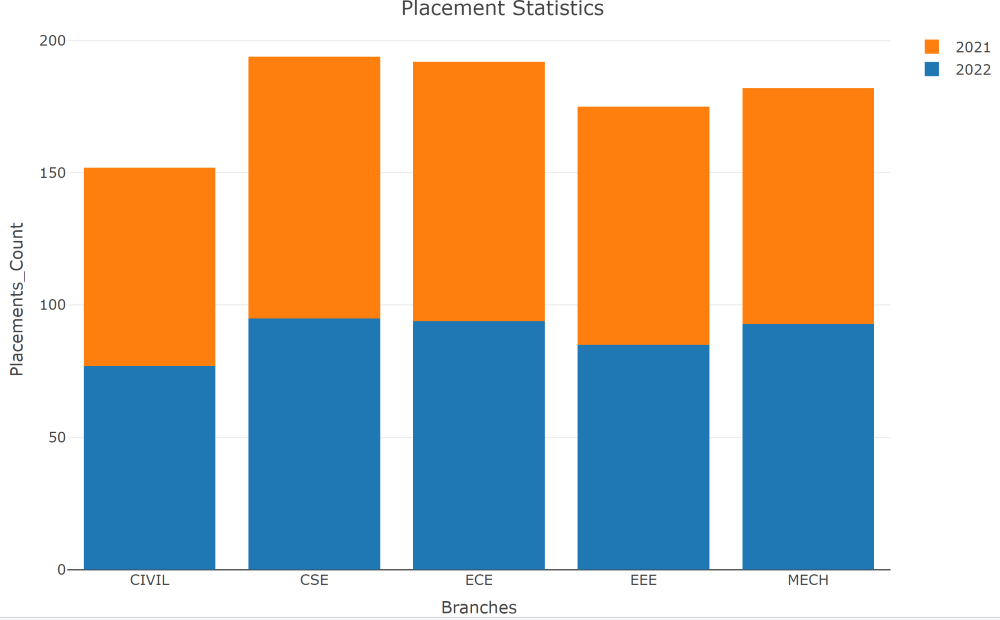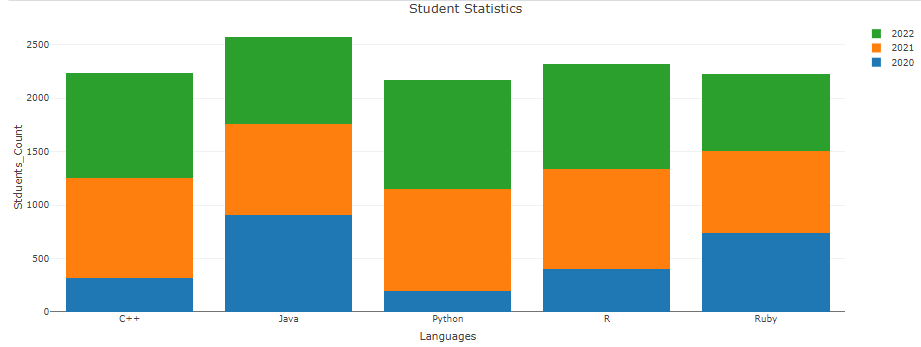Open in App
Not now

# Stacked bar plot Using Plotly package in R

• Difficulty Level : Easy
• Last Updated : 27 Mar, 2023

In general, the bar plots are used to plot the categorical data. The stacked bar plot is a type of bar plot which is used to visualize the data effectively in the same bar by plotting them in a stacked manner. These are mostly used when one wants to summarize similar kinds of data by plotting a single graph. In R Programming the stacked bar plots can be plotted using the plot_ly() function of the plotly package as follows…

Syntax: plot_ly(df,type,marker,labels,values) %>% layout() %>% add_trace()

Where,

• df – data frame
• type – used to specify the type of plot we want to visualize
• marker – used to mark the plot with different colors using color attribute
• labels – names of categorical variables in the dataset
• values – values of the columns in the dataset that we want to plot are specified here (optional)
• layout() –  this function is used to change the layout as required (like assigning a title to the plot)
• add_trace() – this function is used to append similar new traces to existing dimension

Let’s install and load the plotly library with the help of below commands

```install.packages("plotly")
library(plotly)```

### Stack bar plot for two columns

In this, we will be creating a data frame which contains 2 columns and then we will be using plotly module to plot the stacked bar plot.

## R

 `# Creation of sample data frame (Placement Statistics)``data <- ``data.frame``(``  ``Branches<-``c``(``'CSE'``,``'ECE'``,``'MECH'``,``'EEE'``,``'CIVIL'``),``  ``Placements_2021 <-``c``(99,98,89,90,75),``  ``Placements_2022 <- ``c``(95,94,93,85,77))` `# Plotting the stacked bar plot using plot_ly``fig <- plotly::``plot_ly``(data,x = ~Branches,``                       ``y=~Placements_2022, type = ``'bar'``, name = ``'2022'``) %>%``       ``add_trace``(y=~Placements_2021,name = ``'2021'``) %>%``       ``layout``(yaxis = ``list``(title = ``'Placements_Count'``),``              ``barmode = ``'stack'``,title=``"Placement Statistics"``)``fig`

Output:### Stack bar plot for more than two columns

We can also implement the stack bar plotting for more than 2 columns. Let’s see the code for it.

## R

 `# Creation of sample data frame (Student Statistics)``data <- ``data.frame``(``  ``Languages<-``c``(``'Python'``,``'R'``,``'C++'``,``'Java'``,``'Ruby'``),``  ``Batch_2020 <-``c``(199,398,319,910,735),``  ``Batch_2021 <- ``c``(952,942,933,851,771),``  ``Batch_2022 <- ``c``(1022,982,983,811,721))` `# Plotting the stacked bar plot using plot_ly``fig <- plotly::``plot_ly``(data,x = ~Languages,y=~Batch_2020,``                       ``type = ``'bar'``, name = ``'2020'``) %>%``       ``add_trace``(y=~Batch_2021,name = ``'2021'``) %>%  ``        ``add_trace``(y=~Batch_2022,name = ``'2022'``) %>%``       ``layout``(yaxis = ``list``(title = ``'Students_Count'``),``              ``barmode = ``'stack'``,title=``"Student Statistics"``)` `fig`

Output:My Personal Notes arrow_drop_up